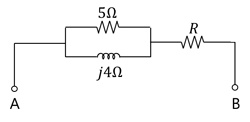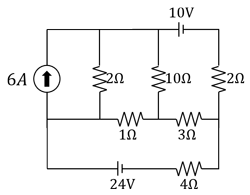MORE IN Basic Electrical & Electronics Engg
MU First Year Engineering (Semester 1)
Basic Electrical & Electronics Engg
December 2011
Total marks: --
Total time: --
INSTRUCTIONS
(1) Assume appropriate data and state your reasons
(2) Marks are given to the right of every question
(3) Draw neat diagrams wherever necessary

1(a) What are the losses in a transformer? Explain why the rating of transformer is in kVA and not in kW
5 M
1(b) Derive the relation between power in Delta and Star Systems
5 M
1(c) A lamp rated 110 Volt, 60 W is connected with another lamp rated 110 Volt, 100 W across 220 volt mains. Calculate the resistance that should be joined in parallel with the first lamp so that both the lamps may take their rated power.
5 M
1(d) Explain the effect of temperature on resistance of different material
5 M

2(a) Explain full wave rectifier using centre tap transformer. Find the expression for RMS and average load current, TUF, rectifier efficiency
10 M
2(b) The voltage of 150V applied between A and B produces a current of 32A. For the circuit shown in figure find the value of R and p.f in the circuit.10 M

3(a) Explain two wattmeter method power measurements in 3 ϕ star connected balanced load.
10 M
3(b) Find the current across 4Ω by superposition theorem.10 M

4(a) Draw and explain input and output characteristics of CE transistor
10 M
4(b) 3Ã¸, 220 V, 50 Hz, 11.2kW Induction motor has full load efficiency of 88% and draws a line current of 38 Amp, under full load, when connected to 3Ã¸, 220 V supply find the reading on two wattmeter connected in the circuit to measure the input to the motor. Determine also p.f. at which motor is operating.
10 M

5(a) An R-L-C series circuit has a current that lags behind the applied voltage by 45o. The voltage across the inductance has maximum value equal to twice the maximum value of voltage across capacitance. The voltage across inductance is 300 sin(1000t) and R = 20Ω. Find the values of inductance and capacitance.
10 M
5(b) Explain double field revolving theory of single phase induction motor
10 M

6(a) 5 kVA , 200/400V , 50 Hz , 1 transformer give following results:
O.C. 200V 0.7A 60W
S.C. 22V 16A 120W
(i) Draw equivalent circuit referred to primary and insert all the parameters
(ii) Find efficiency at 0.9 p.f. at full load.
10 M
6(b) Draw the resonance graph for the following:
(i) XL (ii) R (iii) Z (iv) cos ϕ (v) I
10 M

7(a) Using Norton's theorem, find the current flowing through 100Ω10 M
7(b) Write short notes on the following (any two):